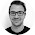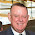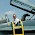## Monday, June 20, 2011

### Fast Approximate Logarithm, Exponential, Power, and Inverse Root

Inspired by Ian Stephenson, I found a faster and more accurate approximation of $\log_2$ which in turns improves my estimates of inverse $p$ roots. The resulting tricks work well generally for computing powers $x^p$ which is also part of computing $L_p$ norm. There's also a fast approximate logarithm and exponential, which are more broadly relevant since these operations are used extensively in practice to represent probabilities.

Update: The code samples here are out of date; minor problems have been fixed and you should get the latest version from the Google code repository. (Update2: Google code is dead, but versions exist on github.)

#### A Fast Approximate Logarithm

A fast $\log_2$ approximation implies a fast approximation for natural log or any other base known at compile time since the difference is one multiply. Therefore, consider a floating-point number $x$ and the value $I_x$ associated with interpreting the byte representation of $x$ as an integer, \begin{aligned} x &= (1 + m_x) 2^{e_x}, \\ \log_2 (x) &= e_x + \log_2 (1 + m_x) ,\\ I_x &= (e_x + B) L + m_x L, \\ I_x / L - B &= e_x + m_x, \\ \log_2 (x) &= I_x / L - B + \log_2 (1 + m_x) - m_x. \end{aligned} Thus what is needed is a way to extract the mantissa from $x$ and approximate $g (z) = \log_2 (1 + z) - z$ for $z \in [0, 1)$. I went with a rational approximation that seemed a sweet spot of accuracy and speed, leading to
// WARNING: this code has been updated.  Do not use this version.

inline float
fastlog2 (float x)
{
union { float f; uint32_t i; } vx = { x };
union { uint32_t i; float f; } mx = { (vx.i & 0x007FFFFF) | (0x7e << 23) };
float y = vx.i;
y *= 1.0 / (1 << 23);

return
y - 124.22544637f - 1.498030302f * mx.f - 1.72587999f / (0.3520887068f + mx.f);
}

inline float
fastlog (float x)
{
return 0.69314718f * fastlog2 (x);
}

If you want even faster and rougher, the average value of $g (z)$ is $\frac{-2 + \log (8)}{\log (4)}$ leading to
// WARNING: this code has been updated.  Do not use this version.

inline float
fasterlog2 (float x)
{
union { float f; uint32_t i; } vx = { x };
float y = vx.i;
y *= 1.0 / (1 << 23);
return y - 126.94269504f;
}

inline float
fasterlog (float x)
{
return 0.69314718f * fasterlog2 (x);
}

##### Timing and Accuracy
Timing tests are done by compiling with -O3 -finline-functions -ffast-math, on a box running 64 bit Ubuntu Lucid (so gcc 4:4.4.3-1ubuntu1 and libc 2.11.1-0ubuntu7.6). I also measured average relative accuracy for $x \in [1/100, 10]$.
fastlog2 relative accuracy = 2.09352e-05
fastlog relative accuracy = 2.09348e-05
fasterlog2 relative accuracy = 0.0130367
fasterlog relative accuracy = 0.0130367
fastlog2 million calls per second = 160.141
fastlog million calls per second = 143.552
fasterlog2 million calls per second = 218.345
fasterlog million calls per second = 210.435
log2f million calls per second = 40.8511


#### A Fast Approximate Exponential

Again 2 is the most convenient base. Here $2^x$ is what I want to approximate and $I_{2^x}$ is the value associated with interpreting the byte pattern of $2^x$ as an integer. \begin{aligned} 2^x &= 2^{x - \lfloor x \rfloor} 2^{\lfloor x \rfloor} \\ &= (1 + 2^{x - \lfloor x \rfloor} - 1) 2^{\lfloor x \rfloor}, \\ I_{2^x} &= (\lfloor x \rfloor + B) L + (2^{x - \lfloor x \rfloor} - 1) L \\ &= L (x + B - 1 + 2^{x - \lfloor x \rfloor} - x + \lfloor x \rfloor). \end{aligned} The correction term is of the form $g (z) = 2^z - z$ for $z \in [0, 1)$, and once again I got the best bang for the buck from a rational function approximation. That leads to
// WARNING: this code has been updated.  Do not use this version.

inline float
fastpow2 (float p)
{
union { float f; uint32_t i; } vp = { p };
int sign = (vp.i >> 31);
int w = p;
float z = p - w + sign;
union { uint32_t i; float f; } v = { (1 << 23) * (p + 121.2740838f + 27.7280233f / (4.84252568f - z) - 1.49012907f * z) };
return v.f;
}

inline float
fastexp (float p)
{
return fastpow2 (1.442695040f * p);
}

If you want even faster and rougher, the average value of $g (z)$ is $\frac{2 - \log (2)}{2 \log (2)}$ leading to
// WARNING: this code has been updated.  Do not use this version.

inline float
fasterpow2 (float p)
{
union { uint32_t i; float f; } v = { (1 << 23) * (p + 126.94269504f) };
return v.f;
}

inline float
fasterexp (float p)
{
return fasterpow2 (1.442695040f * p);
}

##### Timing and Accuracy
Timing tests are done by compiling with -O3 -finline-functions -ffast-math, on a box running 64 bit Ubuntu Lucid (so gcc 4:4.4.3-1ubuntu1 and libc 2.11.1-0ubuntu7.6). I also measured average relative accuracy for $p \in [1/20, 20]$. When I measured relative accuracy I tried each $p$ and also each $p$-th inverse root (i.e., $-1/p$).
fastpow2 relative accuracy (positive p) = 1.58868e-05
fastexp relative accuracy (positive p) = 1.60712e-05
fasterpow2 relative accuracy (positive p) = 0.0152579
fasterexp relative accuracy (positive p) = 0.0152574
fastpow2 relative accuracy (inverse root p) = 1.43517e-05
fastexp relative accuracy (inverse root p) = 1.7255e-05
fasterpow2 relative accuracy (inverse root p) = 0.013501
fasterexp relative accuracy (inverse root p) = 0.0111832
fastpow2 million calls per second = 153.561
fastexp million calls per second = 143.311
fasterpow2 million calls per second = 215.006
fasterexp million calls per second = 214.44
expf million calls per second = 4.16527


#### A Fast Approximate Power

The combination of a fast approximate logarithm and fast approximate exponential yields a fast approximate power.
// WARNING: this code has been updated.  Do not use this version.

inline float
fastpow (float x,
float p)
{
return fastpow2 (p * fastlog2 (x));
}

##### Timing and Accuracy
Timing tests are done by compiling with -O3 -finline-functions -ffast-math, on a box running 64 bit Ubuntu Lucid (so gcc 4:4.4.3-1ubuntu1 and libc 2.11.1-0ubuntu7.6). I also measured average relative accuracy for $(x, p) \in [1/200, 5] \times [1/40, 10]$. When I measured relative accuracy I tried each $p$ and also each $p$-th inverse root (i.e., $-1/p$).
fastpow relative accuracy (positive p) = 0.000165618
fastpow relative accuracy (inverse root p) = 0.00011997
fastpow million calls per second = 58.8533
powf million calls per second = 8.44577


#### A Fast Approximate Inverse Root

By inverse root I mean solving $y = x^{-1/p}$ for $p \geq 1$. Isn't this the same problem as fast approximate power? Yes, but in this regime the following approach is fairly accurate and somewhat faster. The initial approximation is based upon the modified inverse square root hack, $I_y = -\frac{1}{p} I_x + \frac{p + 1}{p} (B - \sigma) L.$ This is refined using approximate Halley's method on $f (y) = \log (y) + \frac{1}{p} \log (x)$, \begin{aligned} y_{n+1} &= y_n \frac{1 - \delta}{1 + \delta} \approx y_n (1 - \delta)^2 \\ \delta &= \frac{\log (2)}{2} \left(\log_2 (y) + \frac{1}{p} \log_2 (x)\right) \end{aligned} The $\log_2$ is computed using the fast approximate logarithm from above. Altogether this becomes
// WARNING: this code has been updated.  Do not use this version.

inline float
fasterinvproot (float x,
float p)
{
union { float f; uint32_t i; } v = { x };
unsigned int R = 0x5F375A84U;   // R = (3/2) (B - sigma) L
unsigned int pR = ((2 * (p + 1)) / (3 * p)) * R;
unsigned int sub = v.i / p;
v.i = pR - sub;
return v.f;
}

inline float
fastinvproot (float x,
float p)
{
float y = fasterinvproot (x, p);
float log2x = fastlog2 (x);
float log2y = fastlog2 (y);
float err = 1.0 - 0.34657359f * (log2x / p + log2y);
return y * err * err;
}

Note: fastinvproot is an improved (faster and more accurate) version of fast_inverse_p_one presented in my previous blog post.
##### Timing and Accuracy
Timing tests are done by compiling with -O3 -finline-functions -ffast-math, on a box running 64 bit Ubuntu Lucid (so gcc 4:4.4.3-1ubuntu1 and libc 2.11.1-0ubuntu7.6). I also measured average relative accuracy for $(x, p) \in [1/200, 5] \times [1/40, 10]$. When I measured relative accuracy I tried each $p$ and also each $p$-th inverse root (i.e., $-1/p$).
fastinvproot relative accuracy (positive p) = 0.000727901
fastinvproot relative accuracy (inverse root p) = 0.00300208
fastinvproot million calls per second = 82.7019

So it's a bit confusing, but the positive p'' case here implies taking an inverse $p$-th root, and inverse root p'' actually means trying to cajole this routine into computing $y = x^p$. Therefore these numbers indicate (for reasons I don't fully understand) fastinvproot is more accurate for finding inverse roots than as a general power routine.

#### Caveat Emptor

In addition to being less accurate, these routines don't handle the floating point special values like the standard routines do. In addition if you feed these routines negative inputs inappropriately, weird stuff happens.

1.Do you know about the Ditch Day stack where ".693147" is the final clue? Pour LN_2 into the tube going in the transom, and the temperature switch opens the door.

2.Your discussion about fast approx pow() reminded me of the classic hack by Schraudolph for very fast exp() by exploiting the IEEE representation. Haven't tested to see how it holds up on modern CPU's vs your approach, but I suspect it would be pretty competitive -- it's basically just 3 FLOPS, with a little clever union-based bit-tweaking, such as:

static union [
double d;
struct [ int j, i; ] n;
] eco;
#define EXPA (1048576/LN(2))
#define EXPC 60801
#define EXP(y) (eco.n.i=EXPA*(y)+(1072693248-EXPC), eco.d)

See: http://cnl.salk.edu/~schraudo/pubs/Schraudolph99.pdf

3.Dennis, I suspect that is a double precision variant of fasterexp presented above, but I haven't dug into the reference you gave.

I have (not presented here) developed a fastsigmoid and fastersigmoid based upon the fastexp and fasterexp functions here. It is interesting that sigmoid (and tanh) tend to reduce the relative error of the approximation due to how they use the exponential; given the reference was motivated by neural networks I could see how he would get away with relatively low precision.

For LDA we needed to approximate lgamma and digamma and I think the additional precision helped.

4.BTW there's an approximation of log_2 mentioned in [Knuth vol. 1]: log_e + log_10. It's accurate to 99%. (Not that it's any use to you!)

5.Wow, that's hard to beat.

5 (log_11 - log_100)

is a better approximation to log_e but is less memorable.

6.Hi Paul,

I have a question about your fast exponential algorithm, fastpow2. It seems to be not very accurate for negative inputs, like for the input -0.01, the your algorithm produces 1.505 whereas the true value should be 0.99

7.@HotLikeAToaster: I just tried this on my machine, and I get:

fastpow2 (-0.01) = 0.993092

8.Thanks so much for all that! I had found the Schraudolph technique that Dennis mentioned up there, but its result can be obviously much improved by a little more sophisticated modeling of the residue with a rational polynomial. I was thinking how I could do it, but this procedure of yours seems to be precisely that. This should be much better known, and available everywhere!...

9.I'm trying to adapt fastexp() to work with double precision, with little success.
I'd appreciate if anyone could point me in the right direction.
Thanks!

1.@DatsMe:

It's not clear if you want something that will work with doubles but with the same precision approximation, or something with higher precision.

The former is easy (if somewhat nonsensical): downcast to float, call the routine, then cast the result back to double.

The latter will require adjusting the constants in the approximation. The mathematica notebook (https://code.google.com/p/fastapprox/source/browse/trunk/fastapprox/tests/fastapprox.nb) contains the derivation which could be adapted to the proper mantissa, base, bitmask, etc. for doubles.

It's possible it will not be faster than standard libraries once you engineer for additional precision.

10.around 1.0 the approximation of fastlog and fasterlog are poor (relative error > 13 for fastlog and much worse for fasterlog)

11.This is also a pretty fast approximation for floats (also is reasonable for double, change log_t to double then), based on a power serie expansion of the arctan identity of ln. The compiler can vectorize it, which speeds it up considerably.

#include
/* Needed for M_LN2 */
#define __USE_MISC 1
#include
#include

/* Predefined constants */
#define i3 0.33333333333333333
#define i5 0.2
#define i7 0.14285714285714285
#define i9 0.11111111111111111
#define i11 0.09090909090909091
#define i13 0.07692307692307693
#define i15 0.06666666666666667

/* alias the type to use the core to make more precise approximations */
typedef float log_t;
/**
This is a logarithmic approximation function. It is done by splitting up the mantissa and the
exponent. And then use the identity ln z = 2 * arctan (z - 1)/(z + 1)
**/

/** Logarithm for small fractions
It calculates ln x, where x <= 1.0
**/
static inline log_t log1ds(log_t z){
log_t pz = (z-1)/(z+1);
log_t pz2 = pz*pz;
log_t pz3 = pz2*pz;
log_t pz5 = pz3*pz2;
log_t pz7 = pz5*pz2;
log_t pz9 = pz7*pz2;
log_t pz11 = pz9*pz2;
log_t pz13 = pz11*pz2;
log_t pz15 = pz13*pz2;
log_t y = 2 * ( pz + pz3 * i3 + pz5 * i5 + pz7 * i7 + pz9 *i9 + pz11 * i11 + pz13 * i13 + pz15 * i15);
return y;
}

#define CALLS 1
// 0000
#define TILL 1000000000
/** The natural logarithm **/
log_t logd(log_t x){
int exp;
log_t fraction = frexpf((log_t)x, &exp);
log_t res = log1ds(fraction);
return res + exp * M_LN2;
}

12.Can we use this for open-source project ?

1.Yes, it is released under the New BSD License.

13.The power series for the exponential converges more rapidly for arguments near zero. So divide your argument by some power of 2 to bring it close to zero, do the truncated power series, and then square the result the appropriate number of times. I don't know if this would beat the stock Exp function, but it is worth a look.

1.It's been a while since I've thought about this, but the bit manipulations are essentially extracting a multiple of a power of two, so that might work better than explicit division and squaring.

14.Thanks for topic. My C sucks (but I am 55 year old lawyer). I used this idea of divide by 2 to make a stab at writing a reasonably accurate and not too slow log function for SIMPOL which doesn't have a built in log function. Once between range of .5 to 1, I worked out some polynomial approximations.
Written is SIMPOL and in a first stab way that I could find my typing and logical errors.

constant log10_2 "0.30102999566398119521373889472449"

function main()
number n
number log
log = 0
integer e
e = 0
string message,title
anyvalue prompt
message = "Entry a number."
title = "log test"
prompt = ""
n = .toval(getuserinput(message,title,prompt, error =e),"",10)
log = jdk_log(n)
end function .tostr(log,10)

function jdk_log(number n)
number log,log10,log3
number eval, shift
integer p
p = 0
eval = n
shift = 0
if n < 1/2
eval = eval * 100
shift = 2
end if

while eval > 1
eval = eval/2
p = p +1
end while
number x, x2, x3,x4, x5, x6, x7, x8, x9, x10
number test10, test3
x = eval
x2 = x * x
x3 = x2 * x
x4 = x3 * x
x5 = x4 * x
x6 = x5 * x
x7 = x6 * x
x8 = x7 * x
x9 = x8 * x
x10 = x9 * x

test10 = -(1436/1000)
test10 = test10 + (5541/1000 * x)
test10 = test10 - (12204/1000 * x2)
test10 = test10 + (13987/1000 * x3)
test10 = test10 + (0304/1000 * x4)
test10 = test10 - (17075/1000 * x5)
test10 = test10 + (4459/1000 * x6)
test10 = test10 + (30204/1000 * x7)
test10 = test10 - (42185/1000 * x8)
test10 = test10 + (23255/1000 * x9)
test10 = test10 - (4849/1000 * x10)

test3 = -(944/1000)
test3 = test3 + (1814/1000 * x)
test3 = test3 - (1241/1000 * x2)
test3 = test3 + (371/1000 * x3)

log10 = p * .toval(log10_2,"",10) + round(test10,1/1000000) - shift
log3 = p * .toval(log10_2,"",10) + round(test3,1/1000000) - shift
log = round((log10 + log3 )/2,1/10000)
end function log

1.Well, I'd never heard of SIMPOL before!

15.FYI: "WARNING: cdn.mathjax.org has been retired. Check https://www.mathjax.org/cdn-shutting-down/ for migration tips."

You might want to update your mathjax cdn, or even switch to KaTex for faster performance! ;)

1.16.Could you please explain what means those magic numbers ((1 << 23), 121.2740838f, 27.7280233f, 4.84252568f, 1.49012907f) in fast exp approximation?

I guess (1 << 23) it is the shift on float mantissa length (23 bits). Am I right?

Thank you!

17.The magic numbers show up when you do a rational function approximation to the residual ( log_2(1+z)-z ).

Yes, the shift is to extract the manitissa: the exponent part of the representation is "easy to log".